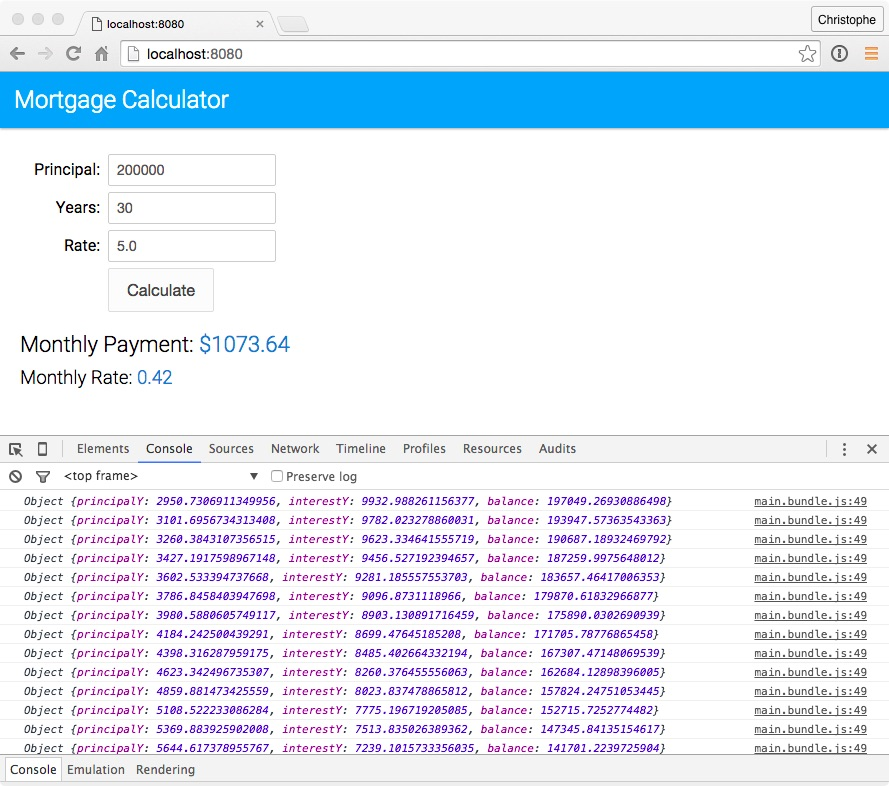# Using Arrow Functions

The ECMAScript 6 arrow function syntax is a shorthand for the ECMAScript 5 function syntax. It supports both block and expression bodies. The value of `this` inside the function is not altered: it is the same as the value of `this` outside the function. No more `var self = this` to keep track of the current scope.

In this unit, you add a new function to calculate the mortgage amortization. You also modify the existing functions to use the new ECMAScript 6 arrow function syntax.

## Steps

1. Open `js/main.js`. Right after the `calculateMonthlyPayment` function, add a `calculateAmortization` function defined as follows:

``````let calculateAmortization = (principal, years, rate) => {
let {monthlyRate, monthlyPayment} = calculateMonthlyPayment(principal, years, rate);
let balance = principal;
let amortization = [];
for (let y=0; y<years; y++) {
let interestY = 0;  //Interest payment for year y
let principalY = 0; //Principal payment for year y
for (let m=0; m<12; m++) {
let interestM = balance * monthlyRate;       //Interest payment for month m
let principalM = monthlyPayment - interestM; //Principal payment for month m
interestY = interestY + interestM;
principalY = principalY + principalM;
balance = balance - principalM;
}
amortization.push({principalY, interestY, balance});
}
return {monthlyPayment, monthlyRate, amortization};
};
``````
2. Modify the `calculateMonthlyPayment` function signature as follows:

``````let calculateMonthlyPayment = (principal, years, rate) => {
``````
3. Modify the signature of the calcBtn click event handler as follows:

``````document.getElementById('calcBtn').addEventListener('click', () => {
``````
4. In the calcBtn click event handler, invoke `calculateAmortization` function instead of `calculateMonthlyPayment`:

``````let {monthlyPayment, monthlyRate, amortization} = calculateAmortization(principal, years, rate);
``````
5. As the last line of the calcBtn click event handler, log amortization data to the console (you’ll display the amortization table in the application in the next unit):

``````amortization.forEach(month => console.log(month));
``````

The complete implementation of the button click handler looks like this:

``````document.getElementById('calcBtn').addEventListener('click', () => {
let principal = document.getElementById("principal").value;
let years = document.getElementById("years").value;
let rate = document.getElementById("rate").value;
let {monthlyPayment, monthlyRate, amortization} = calculateAmortization(principal, years, rate);
document.getElementById("monthlyPayment").innerHTML = monthlyPayment.toFixed(2);
document.getElementById("monthlyRate").innerHTML = (monthlyRate * 100).toFixed(2);
amortization.forEach(month => console.log(month));
});
``````
6. On the command line, type the following command to rebuild the application:

``````npm run babel
``````
7. Open a browser, access http://localhost:8080, and click the Calculate button. Open the developer console: you should see the amortization values in the console log.